• Portfolio
• Stream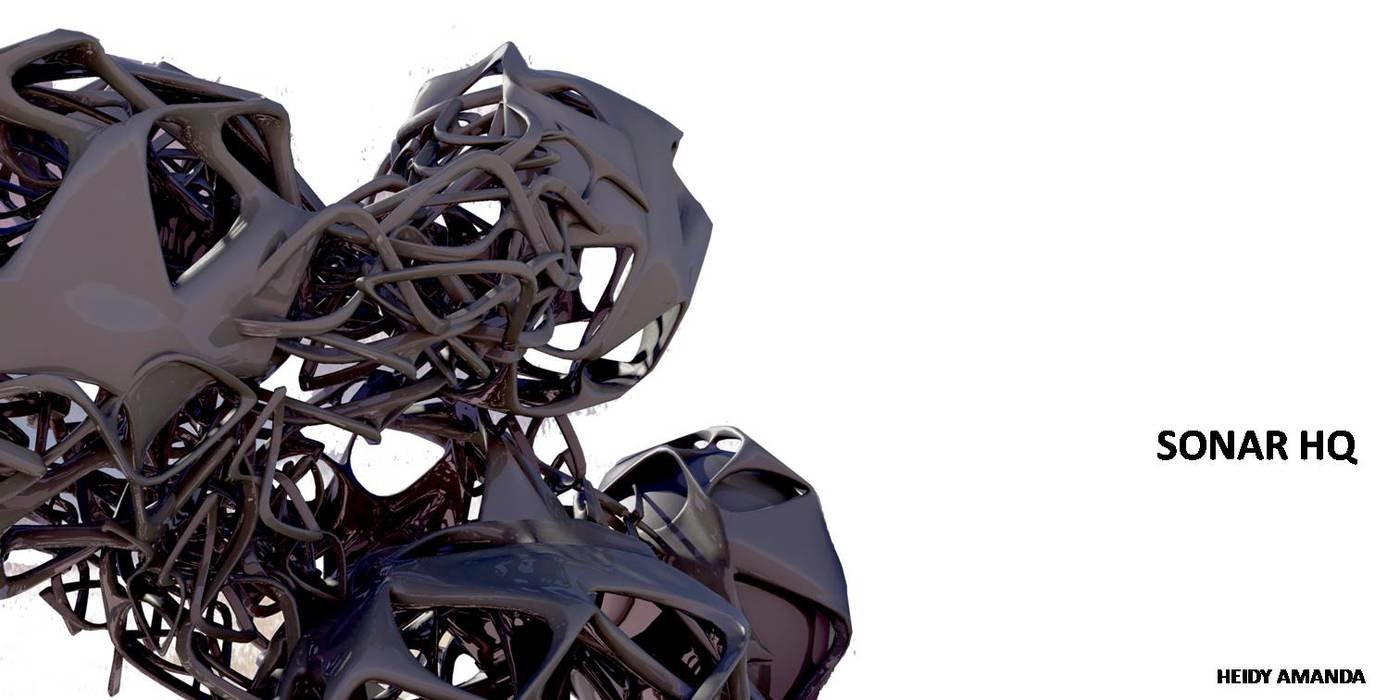Sonar HQ - In this project, there is two terms, the first term is Body and the second one is Vibration.
i took the very essential part of vibration: apogee and perigee. It is translated as a component of the sonar headquarters building which is functioned as apperture. The body of the building itself also take the idea of apogee and perigee. It is composed by the sphere which is connected eachother. After the component(apperture) applied to the body, it will give effect of vibration expression.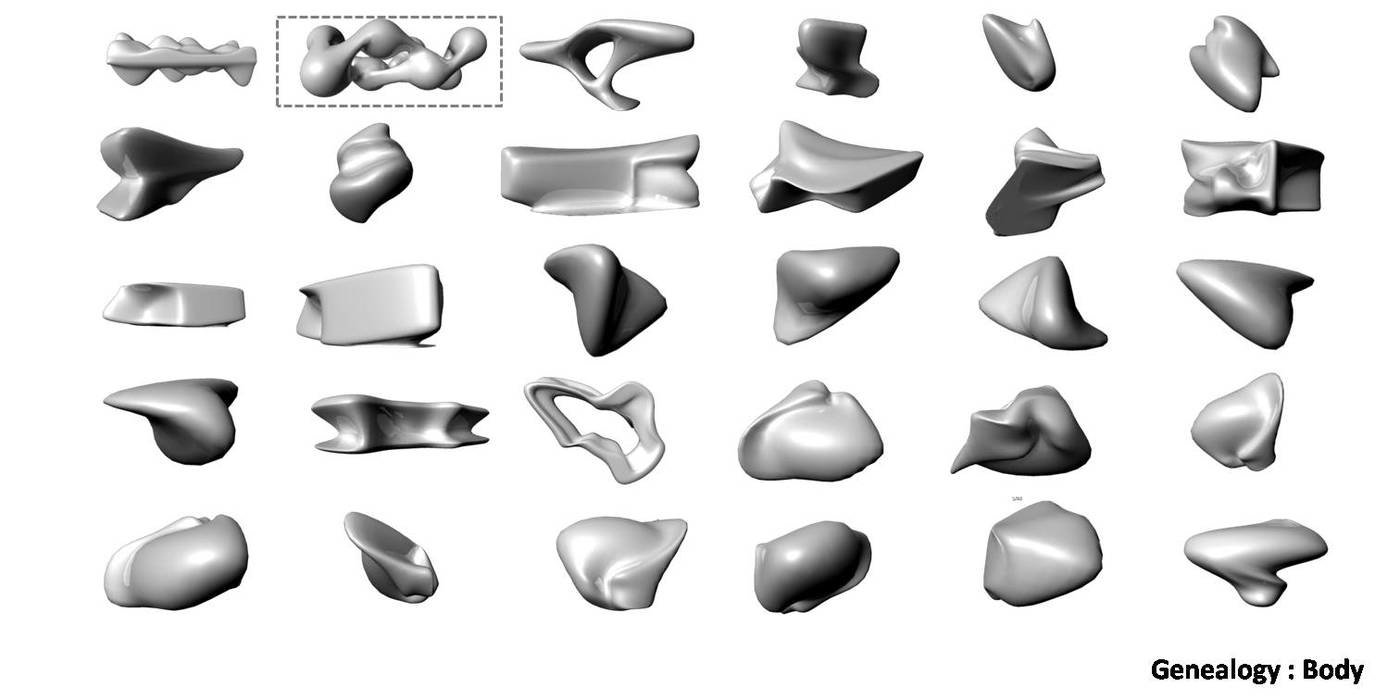Sonar HQ - In this project, there is two terms, the first term is Body and the second one is Vibration.
i to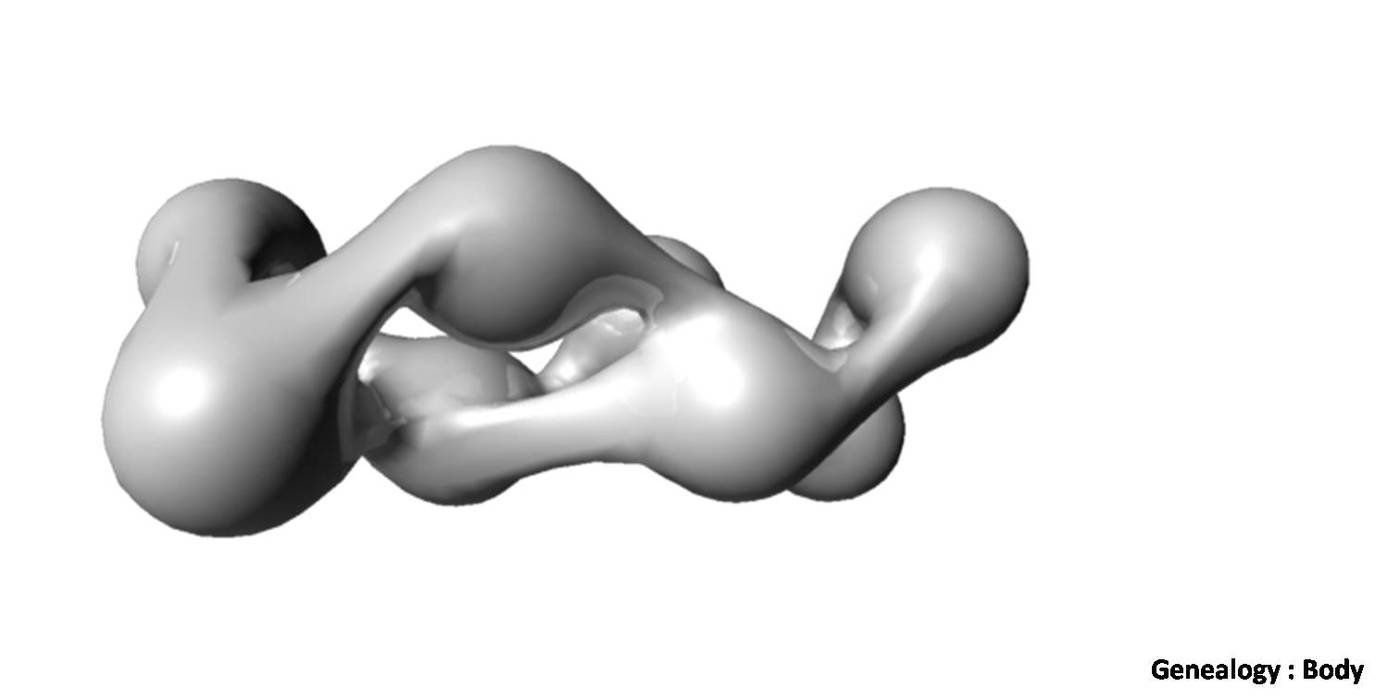Sonar HQ - In this project, there is two terms, the first term is Body and the second one is Vibration.
i to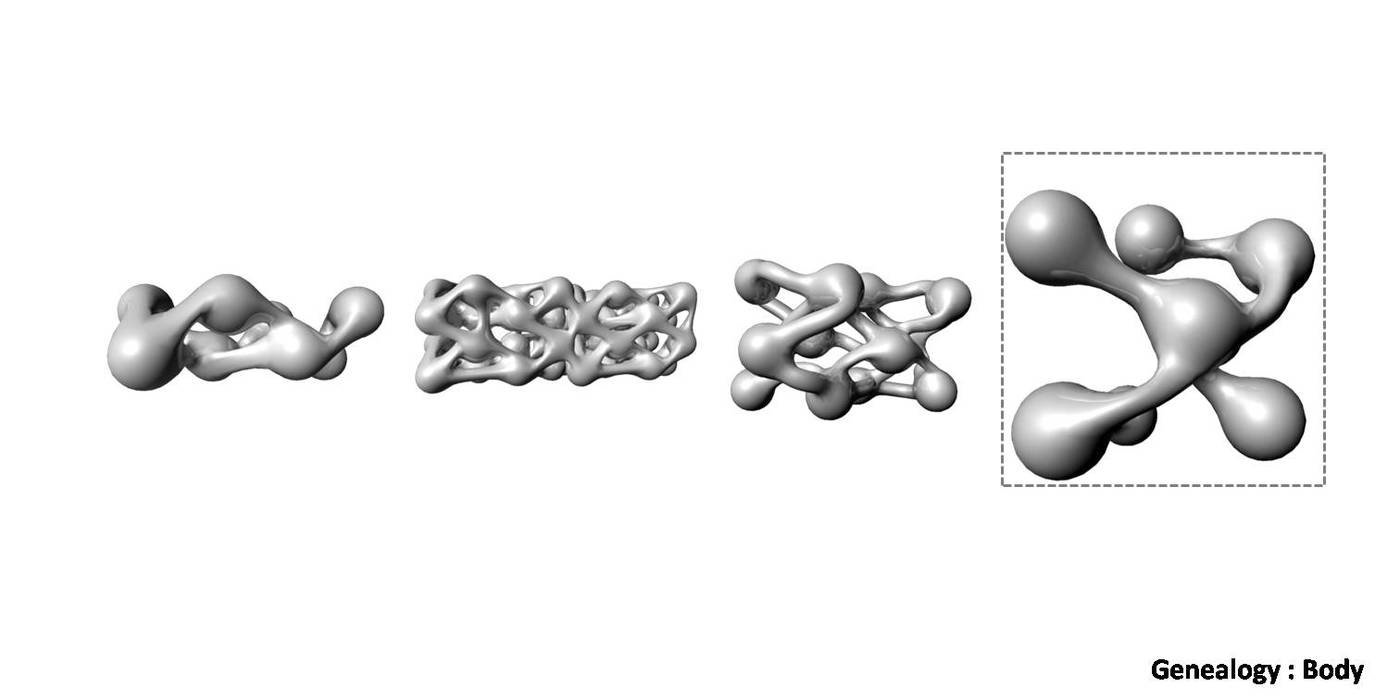Sonar HQ - In this project, there is two terms, the first term is Body and the second one is Vibration.
i to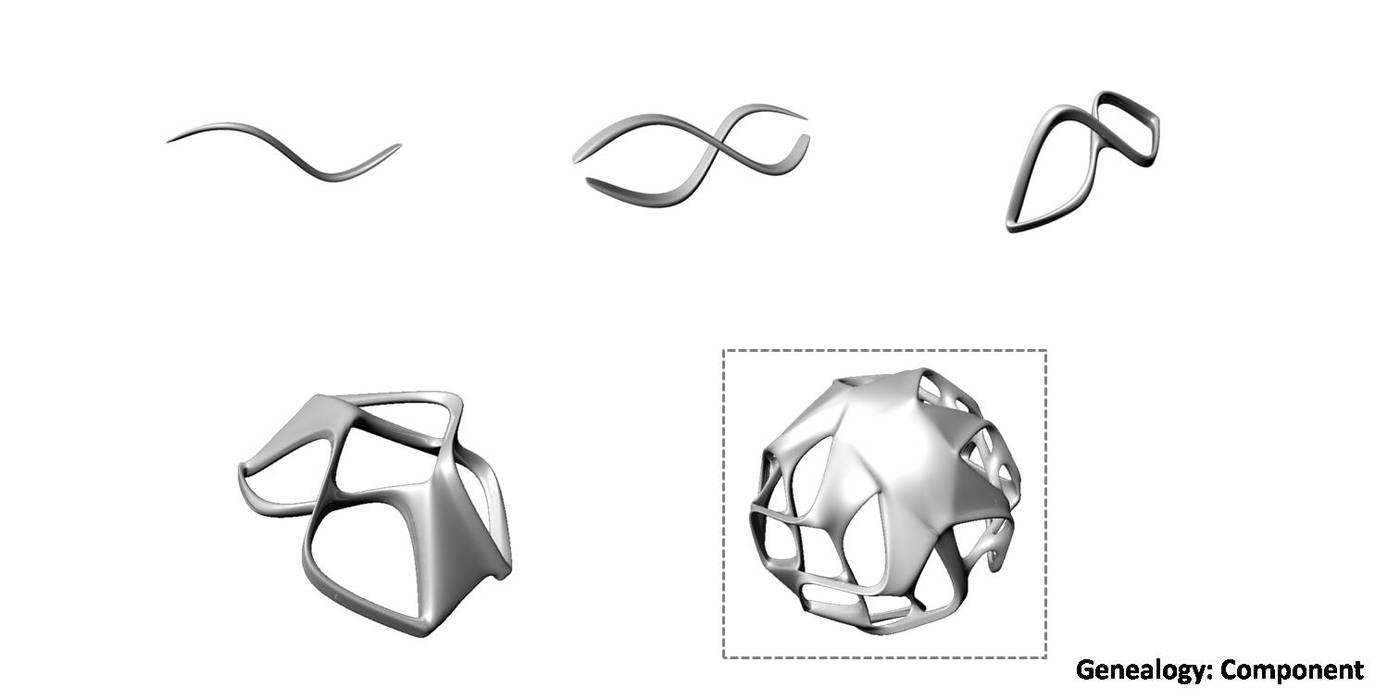Sonar HQ - In this project, there is two terms, the first term is Body and the second one is Vibration.
i to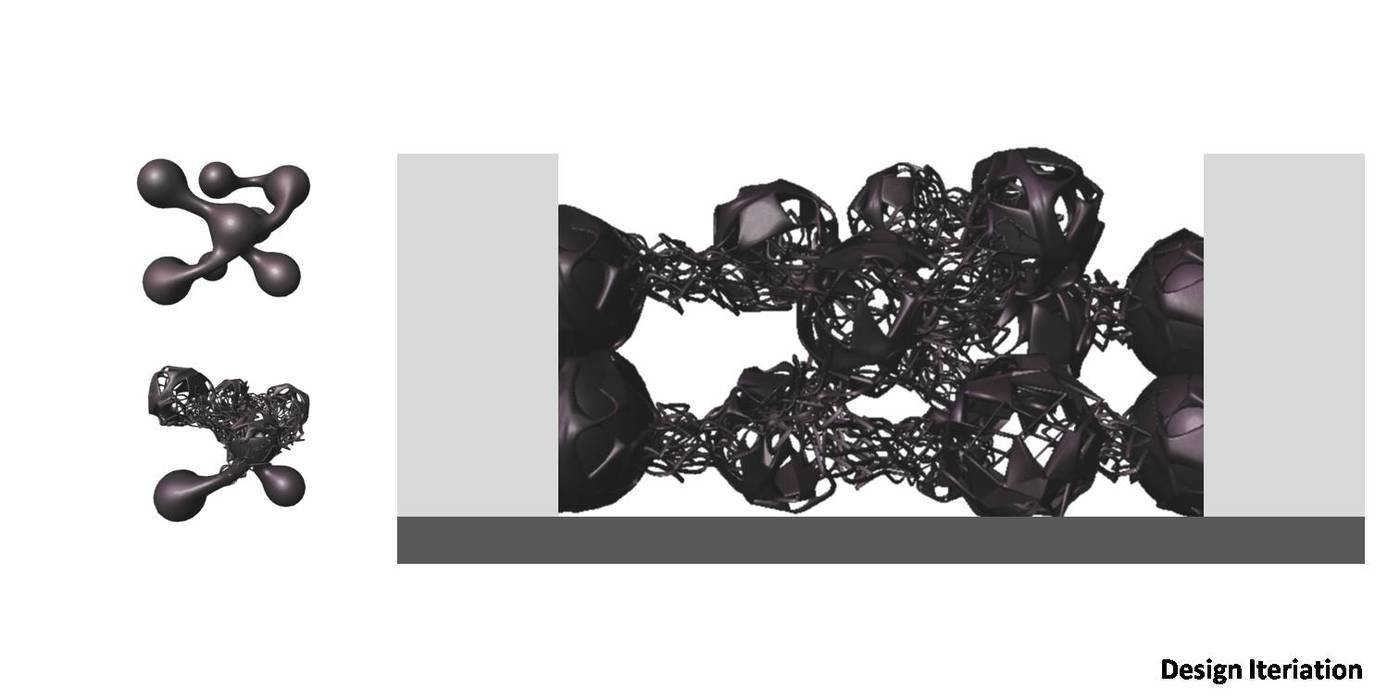Sonar HQ - In this project, there is two terms, the first term is Body and the second one is Vibration.
i to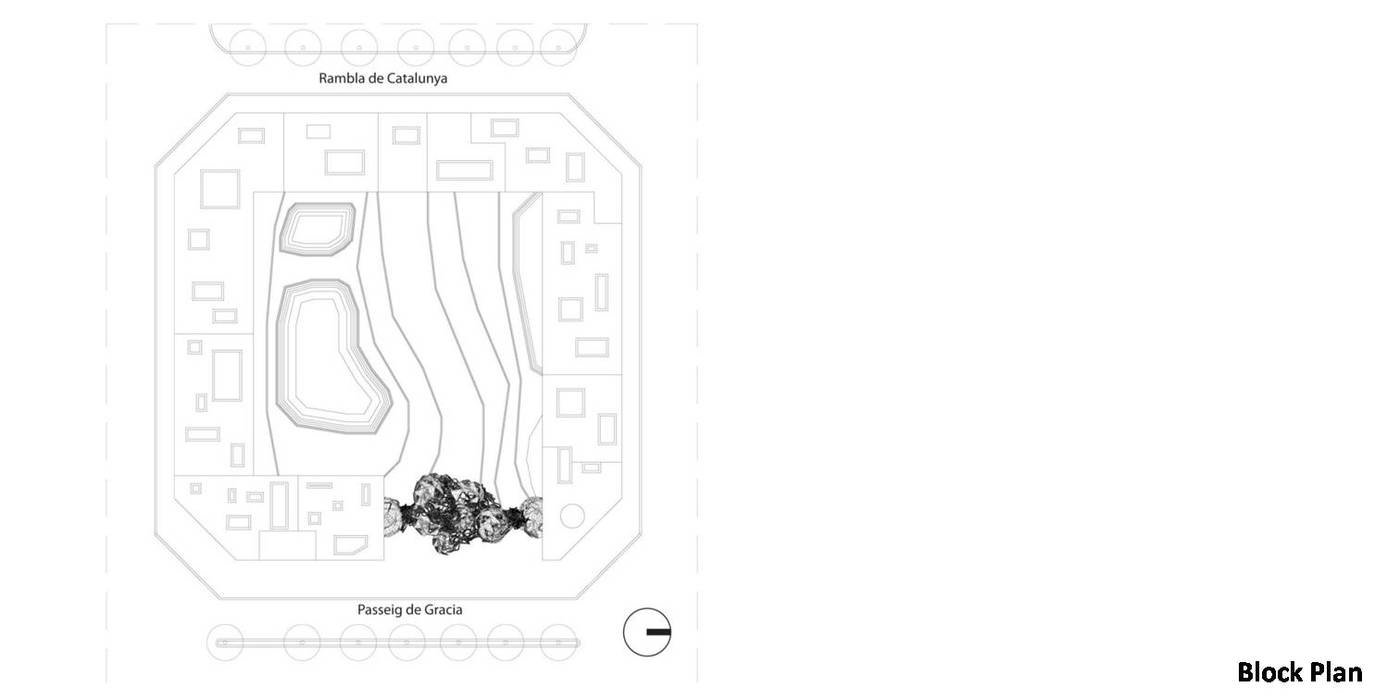Sonar HQ - In this project, there is two terms, the first term is Body and the second one is Vibration.
i to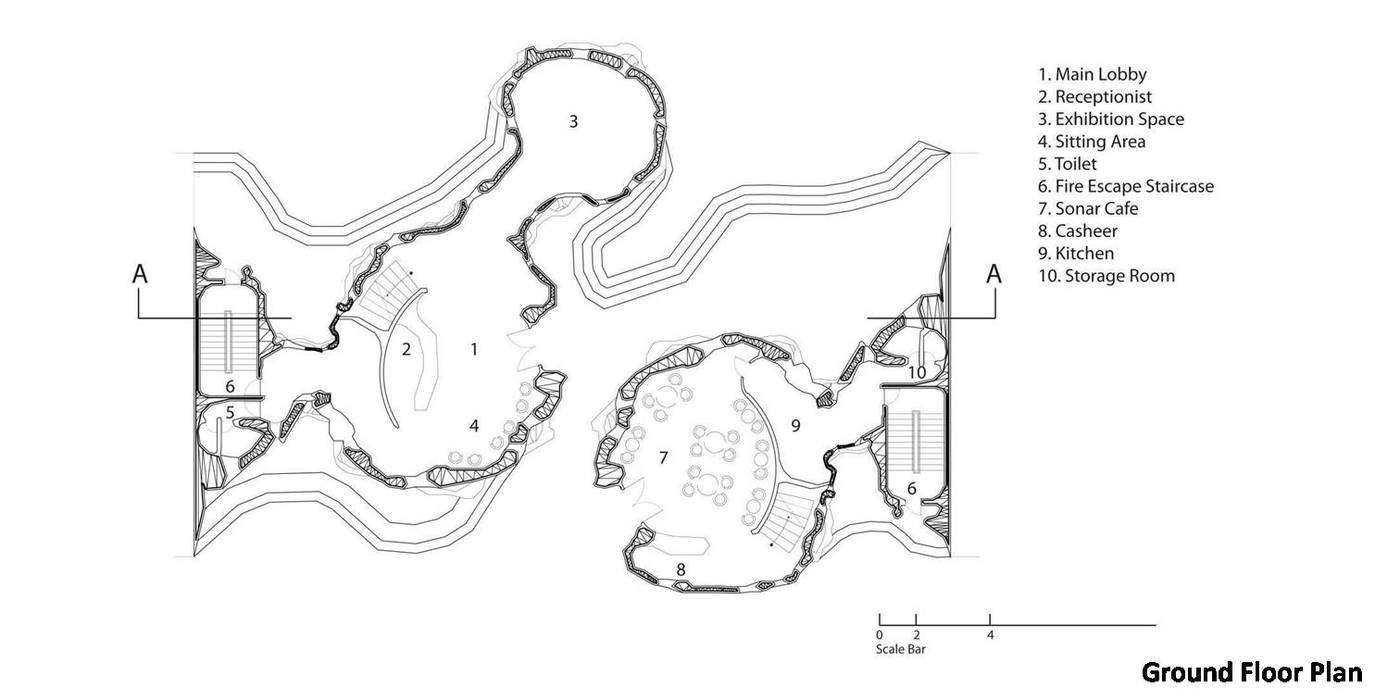Sonar HQ - In this project, there is two terms, the first term is Body and the second one is Vibration.
i to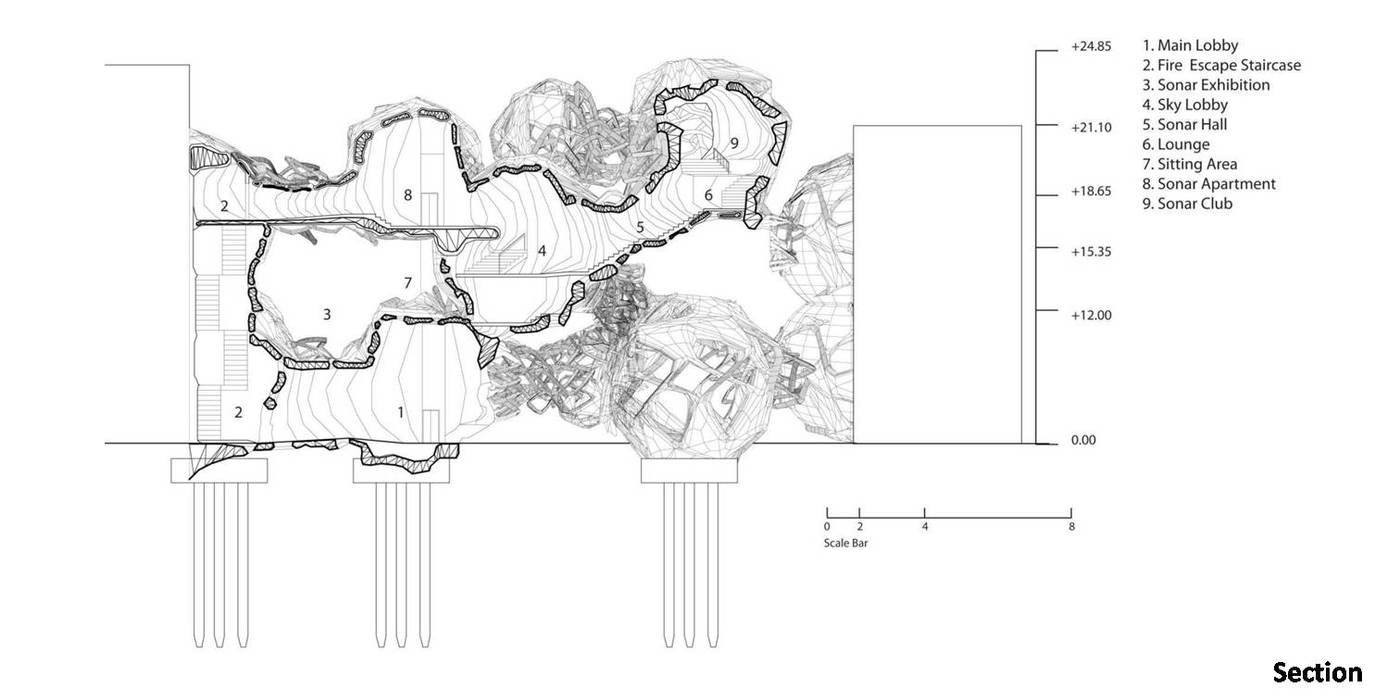Sonar HQ - In this project, there is two terms, the first term is Body and the second one is Vibration.
i to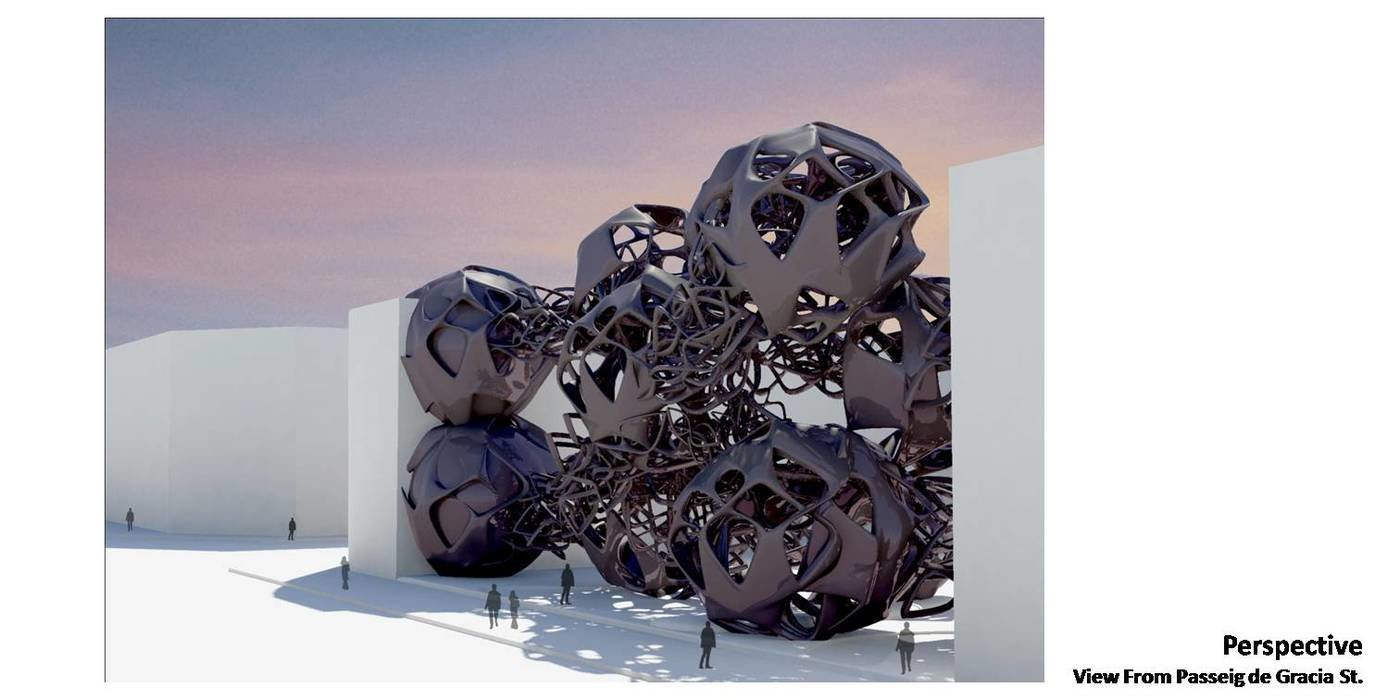Sonar HQ - In this project, there is two terms, the first term is Body and the second one is Vibration.
i to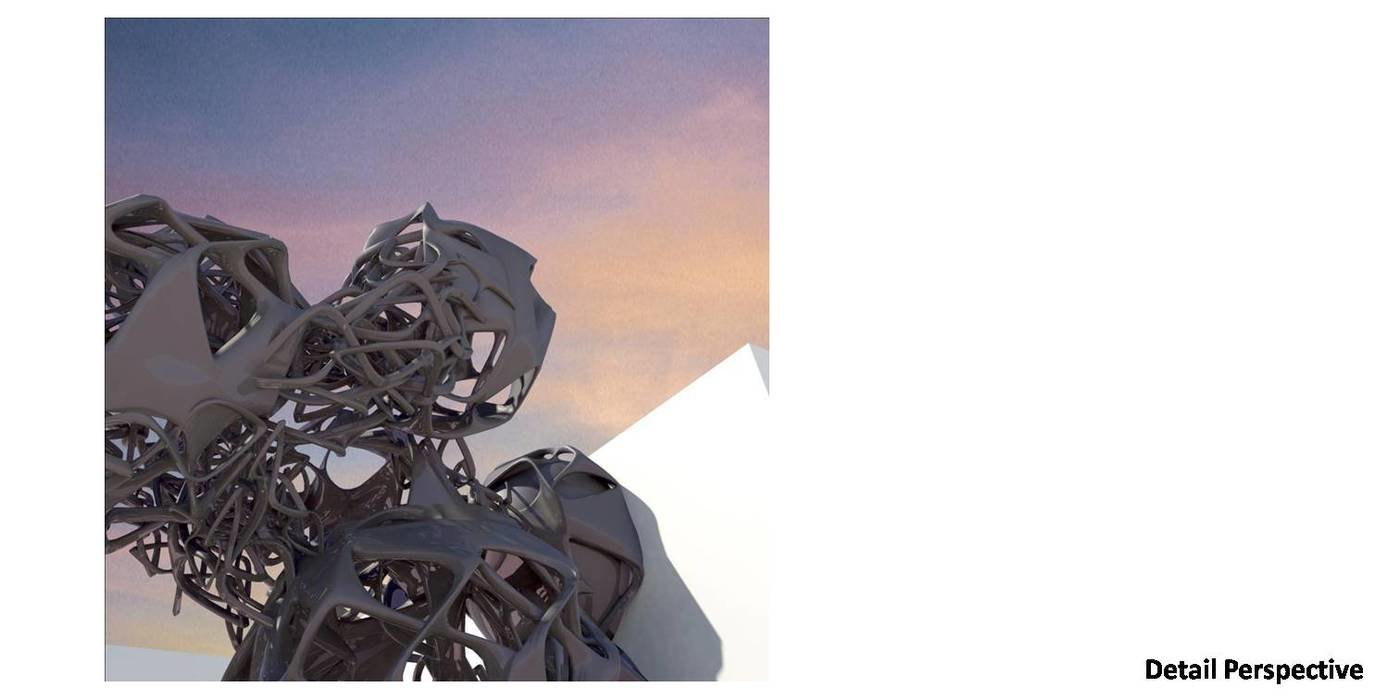Sonar HQ - In this project, there is two terms, the first term is Body and the second one is Vibration.
i to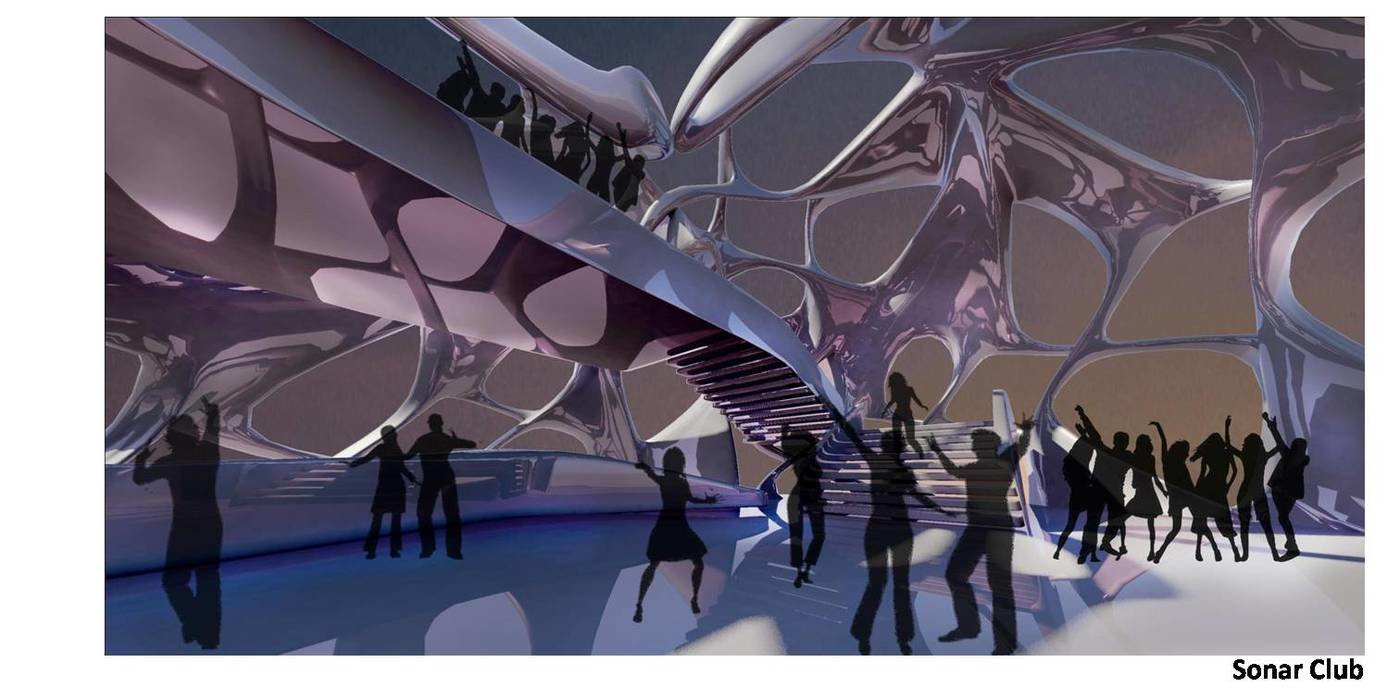Sonar HQ - In this project, there is two terms, the first term is Body and the second one is Vibration.
i to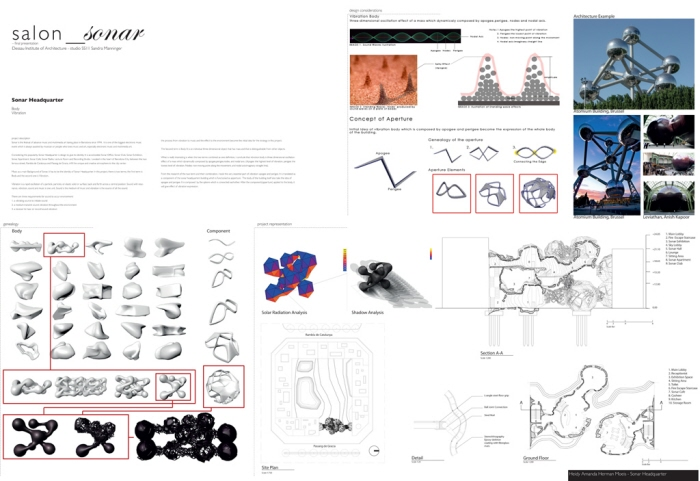Sonar HQ - In this project, there is two terms, the first term is Body and the second one is Vibration.
i to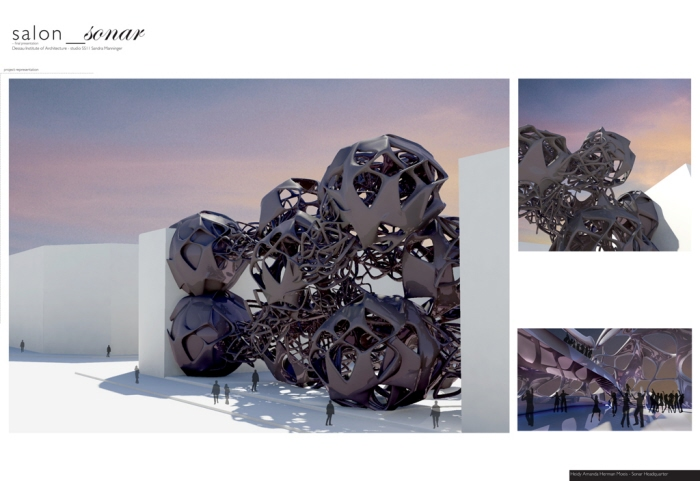Sonar HQ - In this project, there is two terms, the first term is Body and the second one is Vibration.
i to
• o
Salon Sonar Studio
Heidy Amanda Herman...
bandung, Indonesia